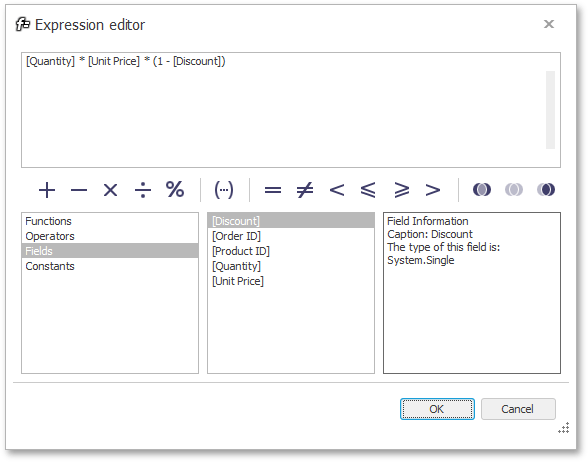20.1
19.2
19.1
18.2
18.1
17.2

# GridColumn.UnboundExpression Property

Gets or sets an expression that the unbound column uses to calculate its cell values. Columns with unbound expressions are non-editable.

Namespace: DevExpress.XtraGrid.Columns

Assembly: DevExpress.XtraGrid.v20.1.dll

## Declaration

``````[DXCategory("Data")]
[DefaultValue("")]
[XtraSerializableProperty]
public string UnboundExpression { get; set; }``````
``````<DXCategory("Data")>
<DefaultValue("")>
<XtraSerializableProperty>
Public Property UnboundExpression As String``````

## Property Value

Type Default Description
String

String.Empty

A string that specifies an expression used to evaluate values for the current column.

## Remarks

Unbound columns can be populated in two ways.

• Specify the UnboundExpression and the column will automatically calculate its cell values. Users are unable to edit cell values if the parent column employs unbound expressions to calculate these values.

• Handle the ColumnView.CustomUnboundColumnData event to provide custom cell values to a column. This approach leaves column cells editable.

At design time, click the ellipsis button (...) next to the UnboundExpression property in the Visual Studio Properties window to invoke the Expression Editor (see the figure below). This editor allows you to build unbound expressions from field names, operators, constants, and mathematical functions. If the GridColumn.ShowUnboundExpressionMenu option is enabled, users can use the same editor at runtime to edit this column's expression.If you need to specify valid expressions in code, refer to the Expressions article for the expression syntax.

## Examples

The following code shows how to create an unbound column (Ext Price) and populate it with data using expressions. Data for this column is calculated according to the formula: [Quantity] * [UnitPrice] * (1 - [Discount]), which is set via the GridColumn.UnboundExpression property.

``````using DevExpress.XtraGrid.Columns;

GridColumn columnExtPrice = new GridColumn();
columnExtPrice.FieldName = "ExtPrice";
columnExtPrice.Caption = "Ext Price";
columnExtPrice.UnboundType = DevExpress.Data.UnboundColumnType.Decimal;
columnExtPrice.UnboundExpression = "[Quantity] * [UnitPrice] * (1 - [Discount])";